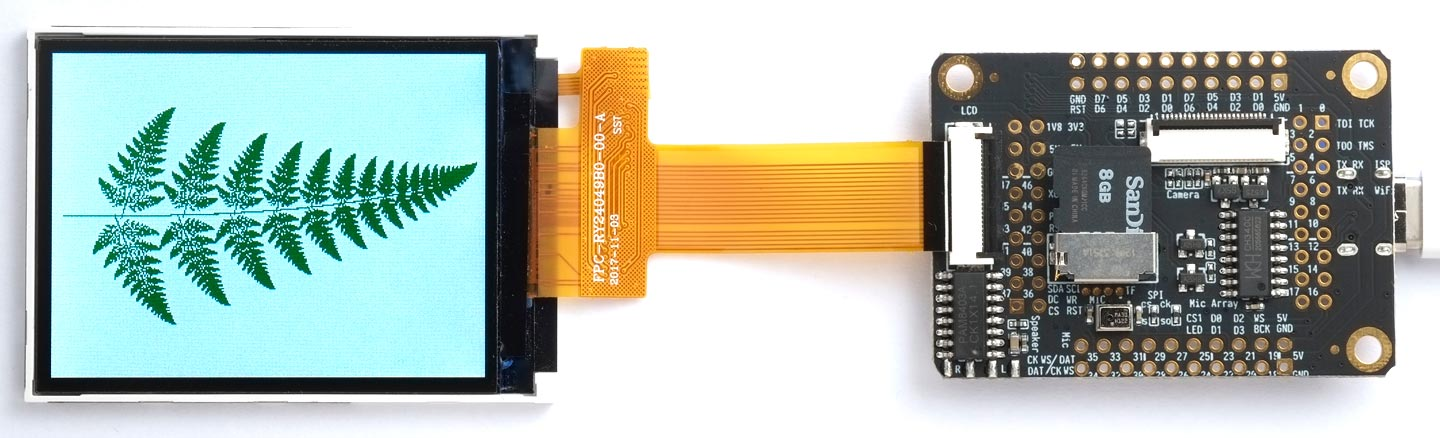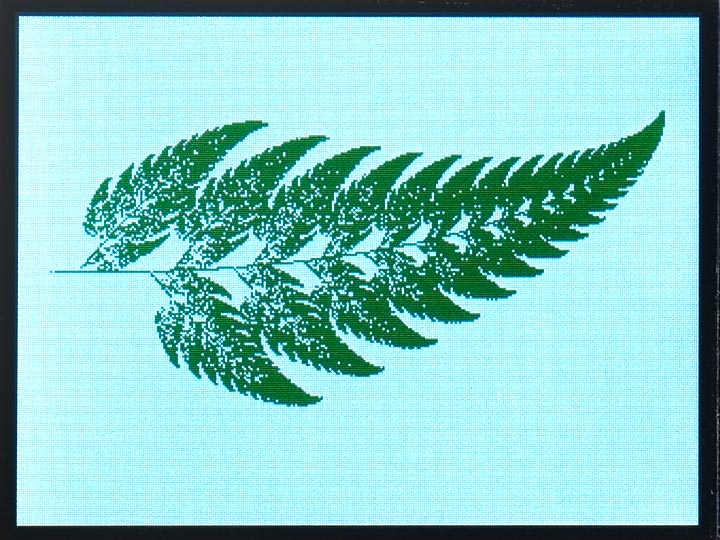## Barnsley Fern

This article describes a uLisp program to plot an intriguing fractal graphic called the Barnsley Fern, that produces leaf shapes like natural ferns:The above photograph shows the program running on a MAiX One Dock, using the uLisp graphics extensions.

### Introduction

The Barnsley Fern  is an Iterated Function System (IFS) created by the British mathematician Michael Barnsley that draws a fractal resembling a fern. It uses four affine transformations that, when called over and over again, transform a single point around the screen.

The transformations are defined in uLisp using a two-dimensional array. For example, the soft bracken fern above (Calochlaena dubia) is generated by the following values designed by David Nicholls :

```(defvar f
#2A((0 0 0 0.25 0 -0.14 0.02)
(0.85 0.02 -0.02 0.83 0 1.0 0.84)
(0.09 -0.28 0.3 0.11 0 0.6 0.07)
(-0.09 0.28 0.3 0.09 0 0.7 0.07)))```

Which of the transformations is picked depends on the last value in each row of the array. So the first transformation is picked with a probability of 0.02, the second with a probability of 0.84, etc.

By altering the numbers you can create fractals with a different appearance. For example, the following values were the ones originally suggested by Michael Barnsley:

```(defvar f
#2A((0 0 0 0.16 0 0 0.01)
(0.85 0.04 -0.04 0.85 0 1.6 0.85)
(0.2 -0.26 0.23 0.22 0 1.6 0.07)
(-0.15 0.28 0.26 0.24 0 0.44 0.07)))

(defvar *factor* (/ *height* 12.5))```

This gives a fern resembling the black spleenwort (Asplenium adiantum-nigrum):Here’s the whole program, suitable for running on a 360x240 display. To run it on a different display change these constants:

```;
; Barnsley Fern

(defvar *width* 240)
(defvar *height* 360)
(defvar *factor* (/ *height* 7))
(defvar *x-offset* (/ *width* 2))
(defvar *y-offset* (/ *height* 24))
(defvar *dark-green* #b0000001111100000)
```

The array f defines the transformations:

```(defvar f
#2A((0 0 0 0.25 0 -0.14 0.02)
(0.85 0.02 -0.02 0.83 0 1.0 0.84)
(0.09 -0.28 0.3 0.11 0 0.6 0.07)
(-0.09 0.28 0.3 0.09 0 0.7 0.07)))```

The program uses these functions:

```(defun fn (n)
#'(lambda (x y)
(list (+ (* (aref f n 0) x) (* (aref f n 1) y) (aref f n 4))
(+ (* (aref f n 2) x) (* (aref f n 3) y) (aref f n 5)))))

(defun choose-transform ()
(let ((r (random 1.0)) (p 0))
(dotimes (i 4)
(when (<= r (incf p (aref f i 6))) (return (fn i))))))

(defun plot-pixel (x y)
(let ((xx (round (+ (* *factor* y) *y-offset*)))
(yy (round (- *width* (+ (* *factor* x) *x-offset*)))))
(draw-pixel xx yy *dark-green*)))```

Finally, here’s the main function:

```(defun fern (&optional (iterations 50000))
(fill-screen #xFFFF)
(let ((x 0) (y 0))
(dotimes (i iterations)
(plot-pixel x y)
(let ((xy (funcall (choose-transform) x y)))
(setq x (first xy))
(setq y (second xy))))))```

To plot the fern call:

`(fern)`

1. ^ Barnsley Fern on Wikipedia.
2. ^ Fractal ferns on Byzantium.